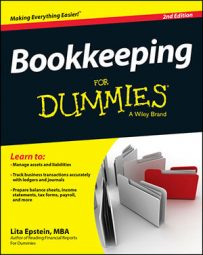##### Bookkeeping For DummiesIn bookkeeping, the acid test or quick ratio evaluates your company’s current assets and liabilities, but it’s a stricter test of cash flow than the similar current ratio. Many lenders prefer the acid test ratio when deciding whether to give you a loan because of that strictness; it doesn’t include the inventory account in the calculation.

Calculating the acid test ratio is a two-step process:

Cash + Accounts Receivable + Marketable Securities = Quick assets

Quick assets ÷ Current liabilities = Quick ratio

The following is an example of an acid test ratio calculation:

\$2,000 + 1,000+ 1,000 = \$4,000 (quick assets)

\$4,000 ÷ \$2,200 = 1.8 (acid test ratio)

Lenders consider a company with an acid test ratio around 1 to be in good condition. An acid test ratio less than 1 indicates that the company may have to sell some of its marketable securities or take on additional debt until it’s able to sell more of its inventory.